Related Articles
Python – Built-in array vs NumPy array
• Last Updated : 17 May, 2020

Let us concentrate on the built-in array module first. Built-in array module defines an object type which can efficiently represent an array of basic values: characters, integers, floating point numbers. Arrays are sequence types and behave very much like lists, except that the type of objects stored in them is constrained.

This means objects stored in the array are of a homogeneous(same) type. Type of objects to be stored in an array (built-in) is determined by typecode. Type codes are single characters.

In array module “array(typecode [, initializer])” returns an array. Where initializer is optional, and if provided it must be a list, string or iterable.

Code:

 `import` `array as built_in ` `array1 ``=` `built_in.array(``'i'``) ` `array1.append(``1``) ` `array1.append(``2``) ` `print``(array1) `

Output:

```array('i', [1, 2])
```

While using built-in array module, it is the call of programmer whether to provide initializer(initializer is optional) during creation of array. On line 2, of the previous code, the typecode was ‘i’ and there we did not provide any initializer and simply appended 1, 2 at the end of the array one by one.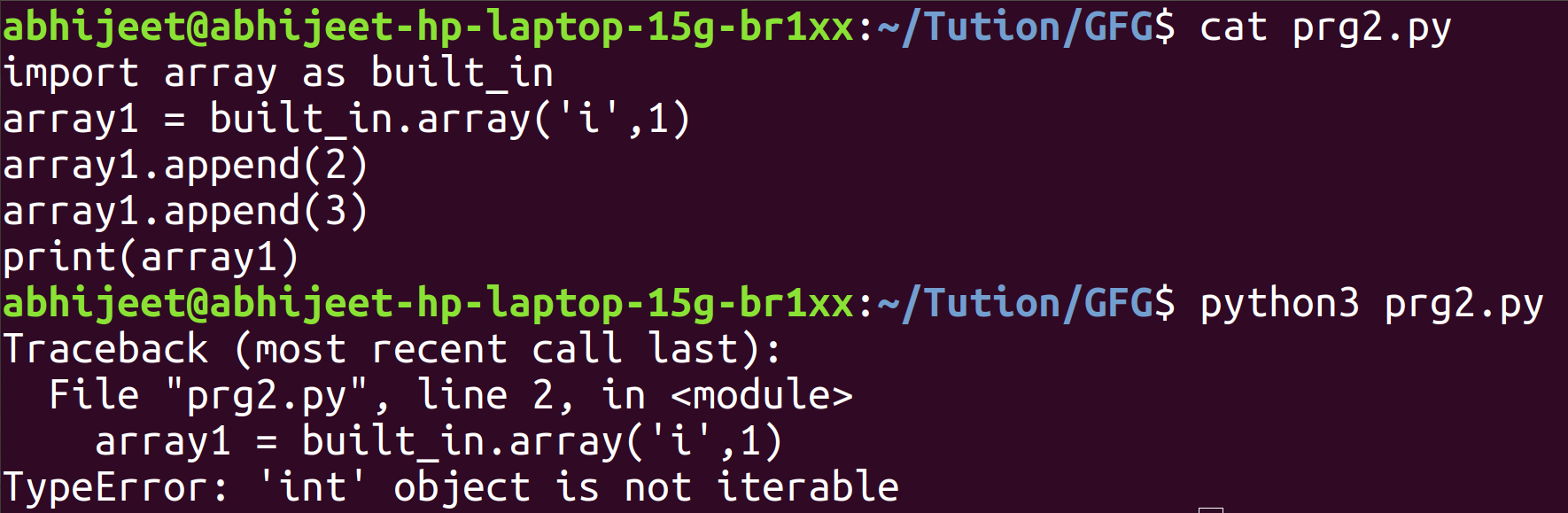Here we did provide an initializer as int value 1. An error occurred because initializer must be iterable and the integer is not iterable.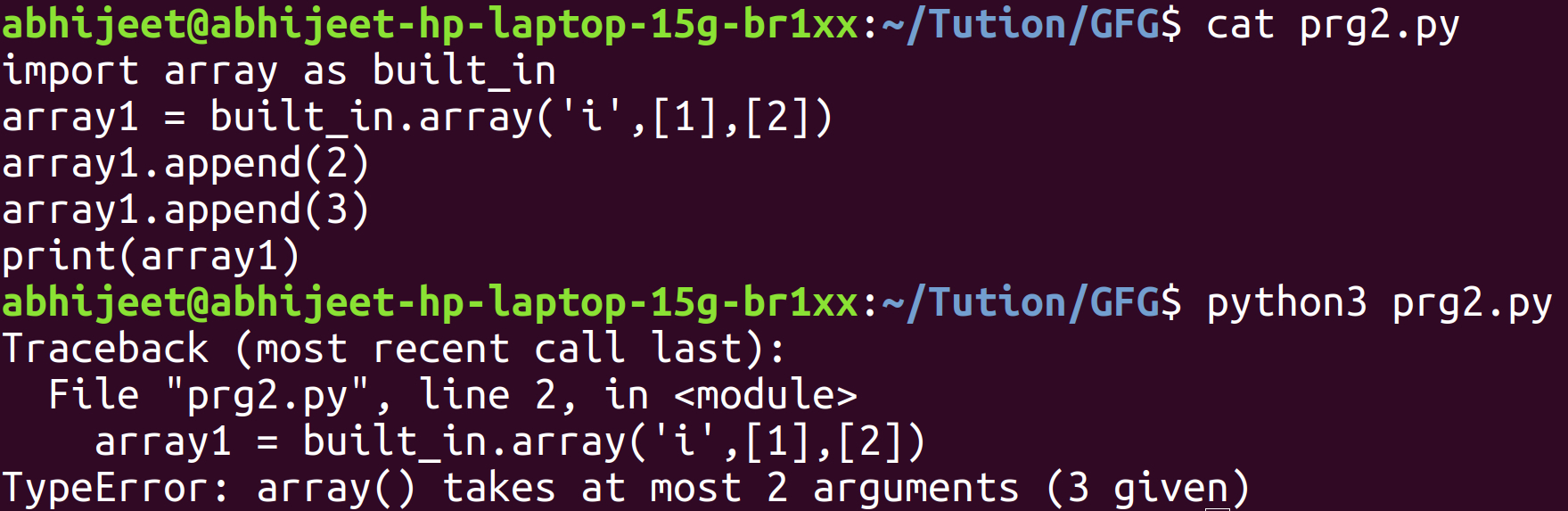Even if we did provide iterable initializers(lists) this time. But array will take 2 arguments at most. And here providing 2 initializers, gives array 3 arguments in total causing a ‘TypeError’.

Code:

 `import` `array as built_in ` `array1 ``=` `built_in.array(``'i'``, [``1``, ``2``]) ` `array1.append(``3``) ` `array1.append(``4``) ` `print``(array1) `

Output:

```array('i', [1, 2, 3, 4])
```

Providing an initializer(at most 1) which is iterable over elements results in error-free execution of the code.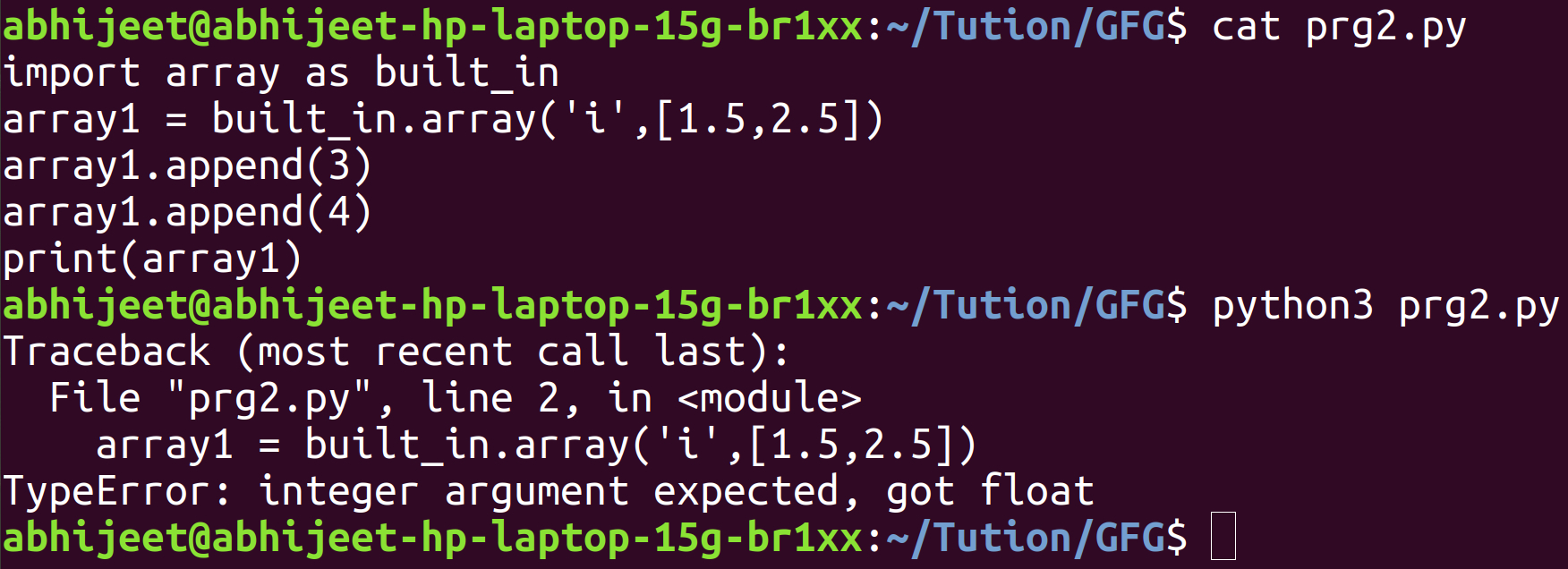Here the typecode given to array was indicating that, objects stored in the array must be of ‘int’ type. Trying to store floating-point value in the array, caused a TypeError.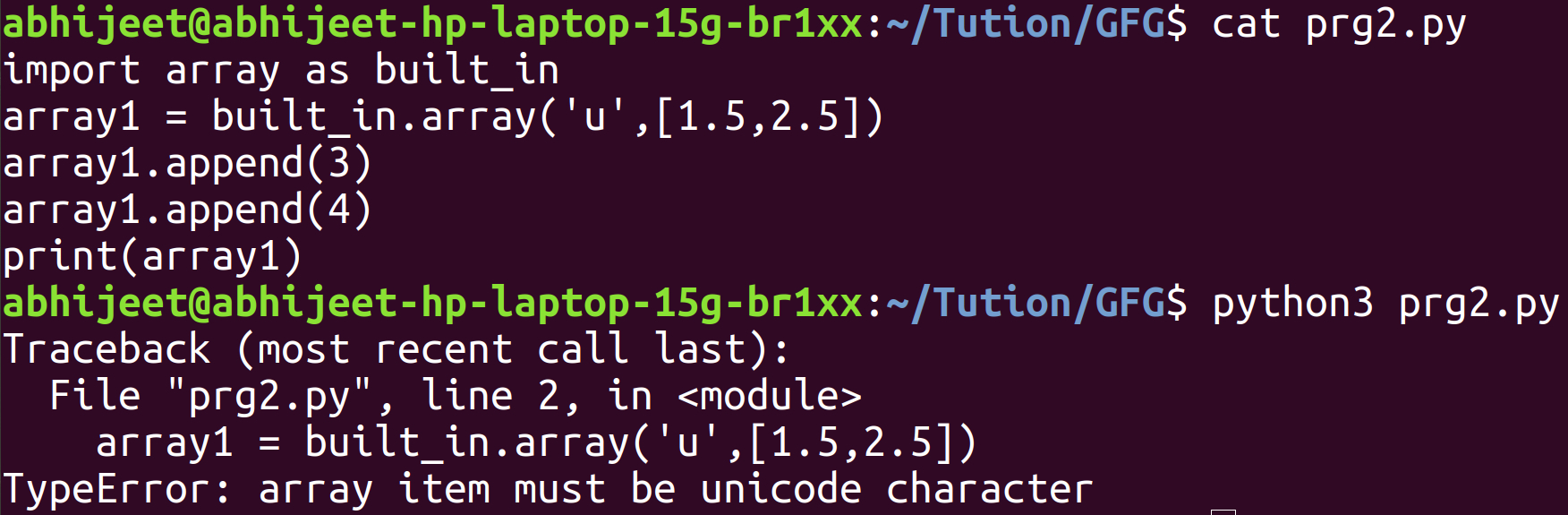Here the typecode given to array was indicating that objects stored in the array must be Unicode character. Trying to store floating-point value in the array, caused a TypeError. Note: built in array module did not typecast data implicitly (from float to int)

Numpy module in python is generally used for matrix and array computations. While using the numpy module, built-in function ‘array’ is used to create an array. A prototype of array function is

array(object, dtype = None, copy = True, order = ‘K’, subok = False, ndmin = 0)

where everything is optional except object. And the object is an array, any object exposing the array interface, an object whose __array__ method returns an array or any (nested) sequence. And dtype is desired data type for array.
Code:

 `import` `numpy ` `array1 ``=` `numpy.array([``1``, ``2``, ``3``]) ` `print``(array1) `

Output:

```[1 2 3]
```

All the other parameters except object, are optional in the array function of numpy module. Thus even if we did not pass other arguments, there was no error.

Code:

 `import` `numpy ` `array1 ``=` `numpy.array([``1``, ``2``, ``3``], ``int``) ` `print``(array1, ``type``(array1[``0``])) `

Output:

```[1 2 3]
```

Here we explicitly told python that, all the objects stored in the array should be typecasted into int(if possible).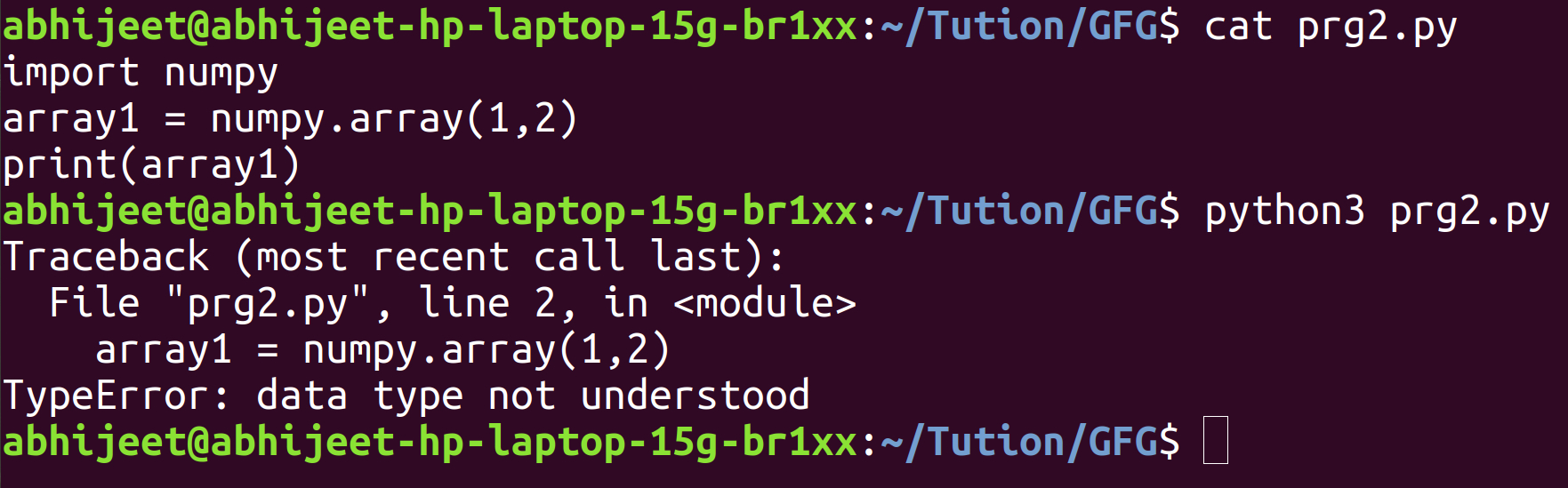Here python took 1 as argument for paramter ‘object’ and 2 as argument for parameter ‘datatype’ and thus python was not able to understand the data type or interpret the data type.

Code:

 `import` `numpy ` `array1 ``=` `numpy.array([``1.5``, ``2.5``], ``int``) ` `print``(array1, ``type``(array1[``0``]), ``type``(array1[``1``])) `

Output:

```[1 2]
```

Here floating point data was typecasted into int, with loss of data after the decimal point, when desired data type of array was int.
Code:

 `import` `numpy ` `array1 ``=` `numpy.array([``1.5``, ``2.5``], ``str``) ` `print``(array1, ``type``(array1[``0``]), ``type``(array1[``1``])) `

Output:

```['1.5' '2.5']
```

Here floating-point data was typecasted into a string. Remember in built-in array module, when the desired datatype of the array was int and float value was passed to the array. A TypeError occurred ‘integer argument expected got float’. And inbuilt array module when the desired data type of array was Unicode character specified by typecode ‘u’, and the floating value was sent to array. TypeError occurred that ‘array item must be Unicode character’. But in numpy array when the desired data type of array was int and float value was sent to array. Float values were typecasted into an int (with loss of data after the decimal) and when the desired data type of array was string and float value were sent to an array, float values were typecasted into a string.
This is the major difference between the built-in array module and numpy array. A built-in array is quite strict about the storage of objects in itself. It permits only that type of data to be stored in itself, which has been specified strictly by the typecode. We could not store float value when typecode specified that, int data has to be stored in the built-in array. However numpy array is a bit tolerant or lenient in that matter, it will upcast or downcast and try to store the data at any cost. (Float was converted to int, even if that resulted in loss of data after decimal)

Note : Built-in array has attributes like typecode and itemsize

typecode — the typecode character used to create the array
itemsize — the length in bytes of one array item

Code:

 `import` `array as built_in ` `array1 ``=` `built_in.array(``'i'``, [``1``, ``2``]) ` `print``(array1) ` `print``(array1.typecode) ` `print``(array1.itemsize) `

Output:

```array('i', [1, 2])
i
4
```

Here the typecode ‘i’ specifies that objects stored in array will be of type signed integer.

Exception: – In numpy array when the desired data type of array is given int or float explicitly and then the string is sent to array. ValueError will occur because it is not really possible to upcast or downcast
string to float or int at any cost.

Attention geek! Strengthen your foundations with the Python Programming Foundation Course and learn the basics.

To begin with, your interview preparations Enhance your Data Structures concepts with the Python DS Course.

My Personal Notes arrow_drop_up
Recommended Articles
Page :